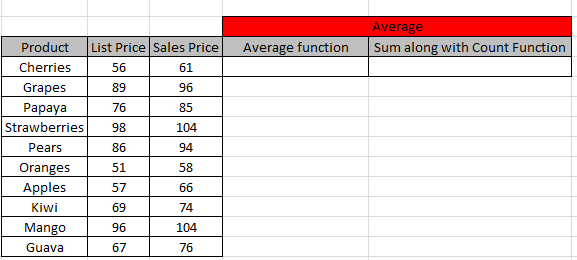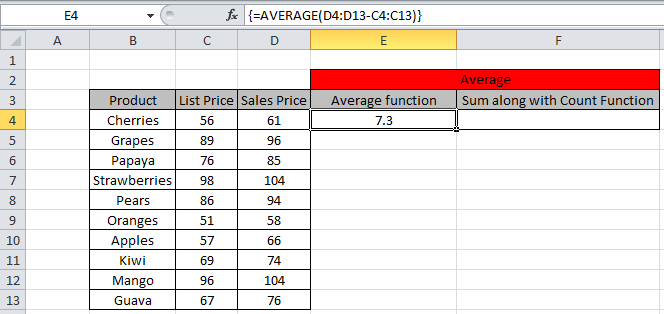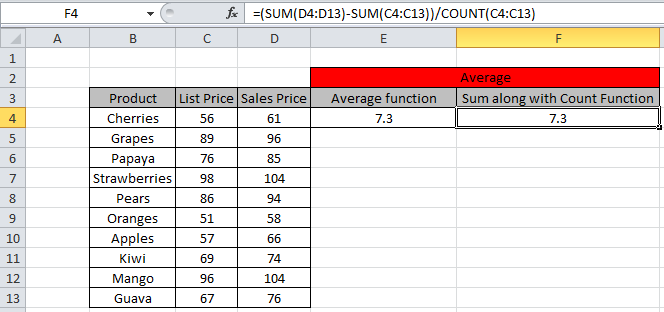# Calculating the Average Difference between List Price and Sale Price in Microsoft Excel

In this article, we will calculate the average difference between the two lists in Excel 2016.
The average difference is the sums of the difference between pairs of consecutive numbers, to get the average, just divide that by the total number of pairs.

All of the above might be confusing for some people, so let’s gear up & start to understand What is average and how we are going to use it in Excel.

Average = (number1 +number2 ..)/ total number of values

We can do the same using the SUM and COUNT function.

SUM function is used to add the values in the list.
Syntax:

=SUM(number1, [number2], ..)

COUNT function is used to count the number of values in the list.
Syntax:

=COUNT(value1, [value2], ..)

Here we have two lists of the products i.e. List Price and Sales Price.We need to find the average difference between the two lists.

We will use the Average formula first

AVERAGE function returns the average (arithmetic mean) of the arguments.

Syntax:

=AVERAGE(number1, [number2], ..)

Use the Formula here.

=AVERAGE(D4:D13 - C4:C13)

Explanation:
This formula calculates the average of D4 to D13 and C4 to C13.
Then it takes the difference between these two.

After writing the formula makes sure to use keyboard keys
Ctrl + Shift + Enter.

To confirm how the above used keyboard keys work, the formula looks like this in fx box in Curly brackets.As shown above the average difference between the two lists.

Use the formula is the dersired cell.

=(SUM(D4:D13) - SUM(C4:C13)) / COUNT(C4:C13)

Explanation:
Sum function gets the sum of D4 to D13 and C4 to C13.
Then we took the difference between the two.
To get the Average difference we will divide it by total number of values using the COUNT function.As we can see, we got the same result from both formulas.

Hope you understood how to use Average function in Excel to find the average difference of the data. You can use these mathematical formulations in Excel 2013 and 2010. Find more articles on mathematical formulation here. Please share your query in the comment box below. We are here to help you.

Popular Articles:

50 Excel Shortcuts to Increase Your Productivity

How to use the VLOOKUP Function in Excel

How to use the COUNTIF function in Excel

How to use the SUMIF Function in Excel

Terms and Conditions of use

The applications/code on this site are distributed as is and without warranties or liability. In no event shall the owner of the copyrights, or the authors of the applications/code be liable for any loss of profit, any problems or any damage resulting from the use or evaluation of the applications/code.